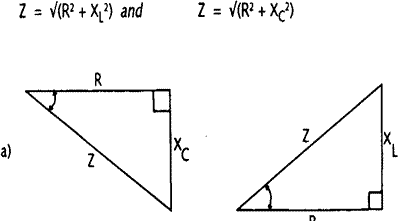16th Edition (reference only) – NOW superseded by the 17th Edition IEE Regulations.
 chapter 1 The IEE Regulations chapter 2 Installation Requirements and Characteristics chapter 3 Installation Control and Protection chapter 4 Cables, Conduits and Trunking chapter 5 Earthing chapter 6 Circuits chapter 7 Special Installations chapter 8 Testing and Inspection chapter 9 Data cabling and Networks
 Earthing
 5.1 - The earthing principle 5.6 - Protective multiple earthing (PME) 5.2 - Earthing Systems 5.7 - Earthed concentric wiring 5.3 - Earth fault loop impedance 5.8 - Other protection methods 5.4 - Protective conductors 5.9 - Residual current devices (RCDs) 5.10 - Combined functional and protective ---------earthing
 5.3.1 - Principle 5.3.2 - The importance of loop impedance 5.3.3 - The resistance/impedance relationship 5.3.4 - Earth-fault loop impedance values 5.3.5 - Protective conductor impedance 5.3.6 - Maximum circuit conductor length

5.3.3 - The resistance/impedance relationship

Resistance, measured in ohms, is the property of a conductor to limit the flow of current through it when a voltage is applied.

 I = U R where I  = current, A U = applied voltage. V R = circuit resistance, Ohms

Thus, a voltage of one volt applied to a one ohm resistance results in a current of one ampere.

When the supply voltage is alternating, a second effect, known as reactance (symbol X) is to be considered. It applies only when the circuit includes inductance and/or capacitance, and its value, measured in ohms, depends on the frequency of the supply as well as on the values of the inductance and/or the capacitance concerned. For almost all installation work the frequency is constant at 50 Hz. Thus, inductive reactance is directly proportional to inductance and capacitive reactance is inversely proportional to capacitance.

 Xl = 2(pi)fL and Xc  = 1 2(pi)fC where Xl = inductive reactance (Ohms) Xc = capacitive reactance (Ohms) (pi) = the mathematical constant (3.142) f = the supply frequency (Hz) L = circuit inductance (H) C = circuit capacitance (F)

Resistance (R) and reactance (Xl or Xc) in series add together to produce the circuit impedance (symbol z), but not in a simple arithmetic manner. Impedance is the effect which limits alternating current in a circuit containing reactance as well as resistance.

 Z = U I where Z  = impedance (Ohms) U = applied voltage (V) I = current (A)

It follows that a one volt supply connected across a one ohm impedance results in a current of one ampere.

When resistance and reactance are added this is done as if they were at right angles, because the current in a purely reactive circuit is 90° out of phase with that in a purely resistive circuit. The relationships between resistance, reactance and impedance are:a) resistive and capacitive circuit - b) resistive and inductive circuit
Fig 5.8 Impedance diagrams

These relationships can be shown in the form of a diagram applying Pythagoras' theorem as shown in {Fig 5.8}. The two diagrams are needed because current lags voltage in the inductive circuit, but leads it in the capacitive. The angle between the resistance R and the impedance Z is called the circuit phase angle, given the symbol a (Greek 'phi'). If voltage and current are both sinusoidal, the cosine of this angle, cos a, is the circuit power factor, which is said to be lagging for the inductive circuit, and leading for the capacitive.

In practice, all circuits have some inductance and some capacitance associated with them. However, the inductance of cables only becomes significant when they have a cross-sectional area of 25 mm² and greater. Remember that the higher the earth fault loop impedance the smaller the fault current will be. Thus, if simple arithmetic is used to add resistance and reactance, and the resulting impedance is low enough to open the protective device quickly enough, the circuit will be safe. This is because the Pythagorean addition will always give lower values of impedance than simple addition.

For example, if resistance is 2 Ohms and reactance 1 Ohm, simple arithmetic addition gives

 Z = R + X – 2 + 1 = 3 Ohms and correct addition gives Z = Ö(R² + X²) = Ö(2² + 1²) = Ö 5 = 2.24 Ohms

If 3 Ohms is acceptable, 2.24 Ohms will allow a larger fault current to flow which will operate the protective device more quickly and is thus even more acceptable.